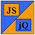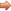# JavaScript & jQuery Tutorials

JS HomeJS ReferenceLogical

## Logical operators

Returns a boolean value where possible or one of the expressions used when not.

### Description

Logical operators are generally used in conditional statement to retrieve a `true` or `false` value and apply this to the conditional statement in question.

• Logical operators are evaluated from left to right.

### `&&` - Logical AND

This operator returns `true` if both its operands can be convered to true otherwise returns `false`.

• If the first operand evaluates to `false` then the operator will 'short circuit' and not test the second operand as both operands have to evaluate to `true`.

Lets see some examples of using the logical AND operator.

``````
// Store properties in an array.
var andProps = new Array(5);
andProps = false && true;
andProps = true && false;
andProps = false && false;
andProps = true && true;
andProps = 'a' && 'b';
``````

Press the button below to action the above code:

### `||` - Logical OR

This operator returns `true` if either of its operands can be convered to true otherwise returns `false`.

• If the first operand evaluates to `true` then the operator will 'short circuit' and not test the second operand as only one operand has to evaluate to `true`.

Lets see some examples of using the logical OR operator.

``````
// Store properties in an array.
var orProps = new Array(5);
orProps = false || true;
orProps = true || false;
orProps = false || false;
orProps = true || true;
orProps = 'a' || 'b';
``````

Press the button below to action the above code:

### `!` - Logical NOT

This operator returns `true` if operand is not true otherwise returns `false`.

Lets see some examples of using the logical NOT operator.

``````
// Store properties in an array.
var notProps = new Array(3);
notProps = !true;
notProps = !false;
notProps = !'a';
``````

Press the button below to action the above code:

## Related Tutorials

JavaScript Intermediate Tutorials - Lesson 3 - Conditional Statements